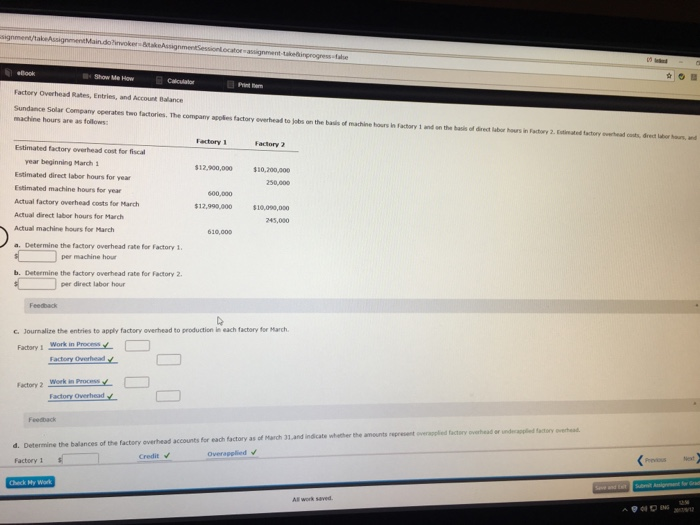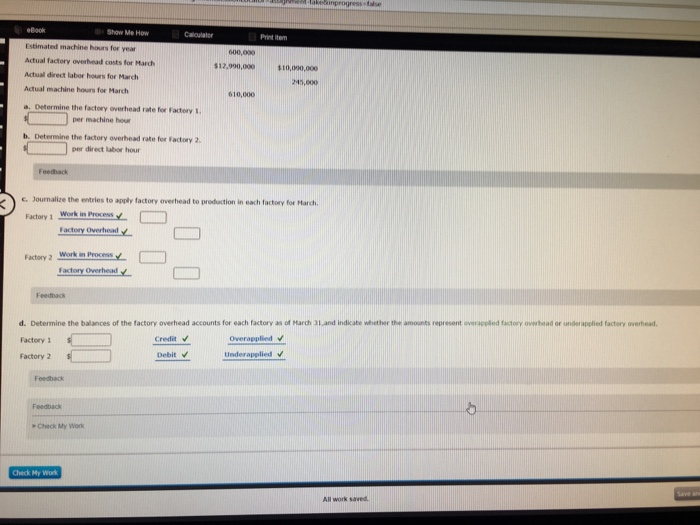# Question & Answer: Show Me Haw Factory Overhead Rates, Entries, and Account Balance Sundance Solar Company operates two factories. The compa…..Solution:

Don't use plagiarized sources. Get Your Custom Essay on
Question & Answer: Show Me Haw Factory Overhead Rates, Entries, and Account Balance Sundance Solar Company operates two factories. The compa…..
GET AN ESSAY WRITTEN FOR YOU FROM AS LOW AS \$13/PAGE

– Factory Overhead Rate is the predetermined overhead rate which is used to apply manufacturing overhead to production department or products or job orders.

– Normally, it is calculated at the beginning of the period.

– It is calculated by dividing the estimated factory overhead cost by an allocation base (or suitable basis).

– Allocation bases may be direct labor hours, direct labor costs, machine hours etc.

Mathematically, FOR is calculated as follows:

Factory Overhead Rate = Estimated Manufacturing Overhead Cost / Estimated Allocation Base

Here in the question, allocation base for factory 1 is Machine Hours and factory 2 is direct labor hours.

(a)

Factory Overhead Rate for factory 1 = Estimated factory Overhead Cost for factory 1/ Estimated Machine Hours for factory 1

= 12,900,000 / 600,000 MHs

= \$21.50 per machine hour

(b)

Factory Overhead Rate for factory 2 = Estimated factory Overhead Cost for factory 2 / Estimated Direct Labor Hours for factory 2

= 10,200,000 / 250,000 DLHs

= \$40.80 per direct labor hour

c)

 Factory 1 (Refer note 1) Work in process 13,115,000 Factory Overhead 13,115,000 Factory 2 (refer note 2) Work in process 9,996,000 Factory Overhead 9,996,000

Note 1 – Calculation of Applied Factory Overhead to production for Factory 1

Factory Overhead Rate (as calculated above) for factory 1 = \$21.50 per machine hour

Actual Machine Hours used for March in factory 1= 610,000 MHs

Applied Factory Overhead = Actual Machine Hours Used x Factory Overhead Rate

= 610,000 MHs x \$21.50 per MH

= \$13,115,000

Note 2 – Calculation of Applied Factory Overhead to production for Factory 2

Factory Overhead Rate (as calculated above) for Factory 2 = \$40.80 per direct labor hour

Actual Direct Labor Hours used for March in factory 2= 245,000 DLHs

Applied Factory Overhead = Actual Direct Labor Hours Used x Factory Overhead Rate

= 245,000 DLHs x \$40.80 per direct labor hour

= \$9,996,000

d)

Factory 1

Over-applied Overhead = 13,115,000 – 12,900,000 = 215,000

Factory 2